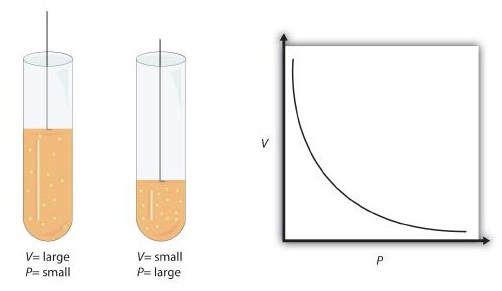# 11.4: Boyle’s Law- Pressure and Volume

Learning Objectives

• Learn what is meant by the term gas laws.
• Learn and apply Boyle’s Law.

When seventeenth-century scientists began studying the physical properties of gases, they noticed some simple relationships between some of the measurable properties of the gas. Take pressure (P) and volume (V), for example. Scientists noted that for a given amount of a gas (usually expressed in units of moles [n]), if the temperature (T) of the gas was kept constant, pressure and volume were related: as one increases, the other decreases. As one decreases, the other increases. This means that pressure and volume are inversely related.

There is more to it, however: pressure and volume of a given amount of gas at constant temperature are numerically related. If you take the pressure value and multiply it by the volume value, the product is a constant for a given amount of gas at a constant temperature:

$P × V = \text{ constant at constant n and T}$

If either volume or pressure changes while amount and temperature stay the same, then the other property must change so that the product of the two properties still equals that same constant. That is, if the original conditions are labeled $$P_1$$ and $$V_1$$ and the new conditions are labeled $$P_2$$ and $$V_2$$, we have

$P_1V_1 = \text{constant} = P_2V_2$

where the properties are assumed to be multiplied together. Leaving out the middle part, we have simply

$P_1V_1 = P_2V_2 \text{ at constant n and T}$

This equation is an example of a gas law. A gas law is a simple mathematical formula that allows you to model, or predict, the behavior of a gas. This particular gas law is called Boyle's Law, after the English scientist Robert Boyle, who first announced it in 1662. Figure $$\PageIndex{1}$$ shows two representations of how Boyle’s Law works.Figure $$\PageIndex{1}$$: Boyle’s Law. A piston having a certain pressure and volume (left piston) will have half the volume when its pressure is twice as much (right piston). One can also plot P versus V for a given amount of gas at a certain temperature; such a plot will look like the graph on the right.

Boyle’s Law is an example of a second type of mathematical problem we see in chemistry—one based on a mathematical formula. Tactics for working with mathematical formulas are different from tactics for working with conversion factors. First, most of the questions you will have to answer using formulas are word-type questions, so the first step is to identify what quantities are known and assign them to variables. Second, in most formulas, some mathematical rearrangements (i.e., algebra) must be performed to solve for an unknown variable. The rule is that to find the value of the unknown variable, you must mathematically isolate the unknown variable by itself and in the numerator of one side of the equation. Finally, units must be consistent. For example, in Boyle’s Law there are two pressure variables; they must have the same unit. There are also two volume variables; they also must have the same unit. In most cases, it won’t matter what the unit is, but the unit must be the same on both sides of the equation.

Example $$\PageIndex{1}$$

A sample of gas has an initial pressure of 2.44 atm and an initial volume of 4.01 L. Its pressure changes to 1.93 atm. What is the new volume if temperature and amount are kept constant?

Solution

Steps for Problem Solving

Identify the "given" information and what the problem is asking you to "find."

Given: P1 = 2.44 atm and V1 = 4.01 L

P2 = 1.93 atm

Find: V2 = ? L

List other known quantities.

none

Plan the problem.

First, rearrange the equation algebraically to solve for $$V_2$$.

$V_2 = \frac{P_1 \times V_1}{P_2}$

Cancel units and calculate.

Now substitute the known quantities into the equation and solve.

$V_2 = \frac{2.44 \: \cancel{\text{atm}} \times 4.01 \: \text{L}}{1.93 \: \cancel{atm}} = 5.07 \: \text{L}$

Think about your result. We know that pressure and volume are inversely related; as one decreases, the other increases. Pressure is decreasing (from 2.44 atm to 1.93 atm), so volume should be increasing to compensate, and it is (from 4.01 L to 5.07 L). So the answer makes sense based on Boyle’s Law.

Exercise $$\PageIndex{1}$$

If P1 = 334 torr, V1 = 37.8 mL, and P2 = 102 torr, what is V2?

124 mL

As mentioned, you can use any units for pressure and volume, but both pressures must be expressed in the same units, and both volumes must be expressed in the same units.

Example $$\PageIndex{2}$$:

A sample of gas has an initial pressure of 722 torr and an initial volume of 88.8 mL. Its volume changes to 0.663 L. What is the new pressure?

Solution

Steps for Problem Solving

Identify the "given" information and what the problem is asking you to "find."

Given: P1 = 722 torr and V1 = 88.8 mL

V2 = 0.633 L

Find: P2 = ? torr

List other known quantities. 1 L = 1000 mL to have the same units for volume.

Plan the problem.

1. Perform the conversion of the second volume unit from L to mL.

2. Rearrange the equation algebraically to solve for $$P_2$$.

$P_2 = \frac{P_1 \times V_1}{V_2}$

Cancel units and calculate.

1. $0.663\, \cancel{L}\times \frac{1000\, ml}{1\, \cancel{L}}=663\, ml$

2. Substitute the known quantities into the equation and solve. $P_2 = \frac{722 \: \text{torr} \times 88.8 \: \cancel{\text{mL}}}{663 \: \cancel{\text{mL}}} = 96.7 \: \text{torr}$

Think about your result. When the volume increased, the pressure decreased, which is as expected for Boyle’s Law.

Exercise $$\PageIndex{2}$$

If V1 = 456 mL, P1 = 308 torr, and P2 = 1.55 atm, what is V2?

119 mL

## Summary

• The behavior of gases can be modeled with gas laws.
• Boyle’s Law relates the pressure and volume of a gas at constant temperature and amount.